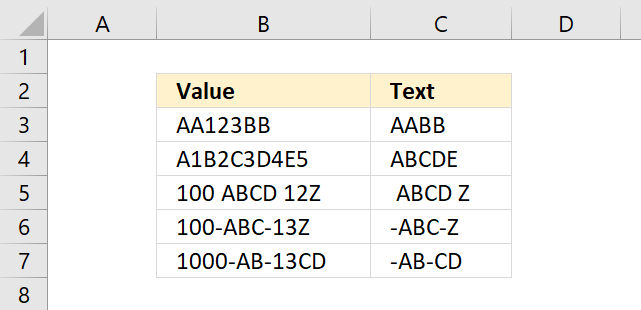Author: Oscar Cronquist Article last updated on January 26, 2022This article demonstrates an array formula that extracts all characters except numbers from a cell, cell C3 contains the formula and it extracts everything except the numbers from cell B3 shown in the image above.

## 1. How to remove numbers from a cell value (Excel 2016)The following formula contains the TEXTJOIN function and it works only in Excel 2016.

=TEXTJOIN("", TRUE, TEXT(MID(B3, ROW(\$A\$1:INDEX(\$A\$1:\$A\$1000, LEN(B3))), 1), ""))

It allows you to filter values up to 1000 characters and you can easily change that limit by changing this cell reference: \$A\$1:\$A\$1000 in the formula above.

### Explaining the Excel 2016 array formula in cell C3

#### Step 1 - Count characters in cell B3

The LEN function returns the number of characters in a cell value.

LEN(text)

LEN(B3)

becomes

LEN("AA123BB")

and returns 7.

#### Step 2 - Create a reference

The INDEX function returns a value based on a row and column number (optional), however, it can also be used to create a dynamic cell reference.

INDEX(\$A\$1:\$A\$1000, LEN(B3))

becomes

INDEX(\$A\$1:\$A\$1000, 7)

and returns A7.

#### Step 3 - Create a cell reference to a cell range

\$A\$1:INDEX(\$A\$1:\$A\$1000, LEN(B3))

becomes

\$A\$1:A7

#### Step 4 - Create row numbers based on cell ref

The ROW function returns a number representing the row number of a given cell reference.

ROW(\$A\$1:INDEX(\$A\$1:\$A\$1000, LEN(B3)))

becomes

ROW(A1:A7)

and returns

{1; 2; 3; 4; 5; 6; 7}.

#### Step 5 - Split characters in cell B3

The MID function returns a substring from a string based on the starting position and the number of characters you want to extract.

MID(textstart_numnum_chars)

MID(B3, ROW(\$A\$1:INDEX(\$A\$1:\$A\$1000, LEN(B3))), 1)

becomes

MID("AA123BB", {1; 2; 3; 4; 5; 6; 7}, 1)

and returns

{"A"; "A"; "1"; "2"; "3"; "B"; "B"}.

#### Step 6 - Remove numbers from array

The TEXT function lets you apply formatting to a given value.

TEXT(valueformat_text)

TEXT(MID(B3, ROW(\$A\$1:INDEX(\$A\$1:\$A\$1000, LEN(B3))), 1), "")

becomes

TEXT({"A"; "A"; "1"; "2"; "3"; "B"; "B"}, "")

and returns

{"A"; "A"; ""; ""; ""; "B"; "B"}.

#### Step 7 - Join remaining characters

The TEXTJOIN function concatenates values in a cell range or array.

TEXTJOIN(delimiterignore_emptytext1[text2], ...)

TEXTJOIN("", TRUE, TEXT(MID(B3, ROW(\$A\$1:INDEX(\$A\$1:\$A\$1000, LEN(B3))), 1), ""))

becomes

TEXTJOIN("", TRUE, {"A"; "A"; ""; ""; ""; "B"; "B"})

and returns "AABB".

## 2. How to remove numbers from a cell value (Excel 365)Update 1/12/2021 new dynamic array formula in cell C3:

=TEXTJOIN("", TRUE, TEXT(MID(B3, SEQUENCE(LEN(B3)), 1), ""))

This formula works only with Excel 365, it contains the new SEQUENCE function that creates an array containing numbers from 1 to n.

### 2.1 Explaining the Excel 365 dynamic array formula in cell C3

#### Step 1 - Count characters in cell B3

The LEN function returns the number of characters in a cell value.

LEN(text)

LEN(B3)

becomes

LEN("AA123BB")

and returns 7.

#### Step 2 - Create an array of numbers from 1 to n

The SEQUENCE fucntion creates a list of sequential numbers to a cell range or array. It is located in the Math and trigonometry category and is only available to Excel 365 subscribers.

SEQUENCE(rows, [columns], [start], [step])

SEQUENCE(LEN(B3))

becomes

SEQUENCE(7)

and returns

{1; 2; 3; 4; 5; 6; 7}.

#### Step 3 - Split value into characters

The MID function returns a substring from a string based on the starting position and the number of characters you want to extract.

MID(textstart_numnum_chars)

MID(B3, SEQUENCE(LEN(B3)), 1)

becomes

MID("AA123BB", {1; 2; 3; 4; 5; 6; 7}, 1)

and returns

{"A"; "A"; "1"; "2"; "3"; "B"; "B"}.

#### Step 4 - Remove numbers in the array

The TEXT function lets you apply formatting to a given value.

TEXT(valueformat_text)

TEXT(MID(B3, SEQUENCE(LEN(B3)), 1), "")

becomes

TEXT({"A"; "A"; "1"; "2"; "3"; "B"; "B"}, "")

and returns

{"A"; "A"; ""; ""; ""; "B"; "B"}.

#### Step 5 - Join remaining characters

The TEXTJOIN function concatenates values in a cell range or array.

TEXTJOIN(delimiterignore_emptytext1[text2], ...)

TEXTJOIN("", TRUE, TEXT(MID(B3, SEQUENCE(LEN(B3)), 1), ""))

becomes

TEXTJOIN("", TRUE, {"A"; "A"; ""; ""; ""; "B"; "B"})

and returns "AABB".